﻿ 城市复杂环境下GNSS/INS组合导航算法研究文章快速检索 高级检索
 大地测量与地球动力学2022, Vol. 42Issue (1): 15-20  DOI: 10.14075/j.jgg.2022.01.004### 引用本文WANG Fu, HAN Baomin, HU Liangliang, et al. Research on GNSS/INS Integrated Navigation Algorithm in Complex Urban Environments[J]. Journal of Geodesy and Geodynamics, 2022, 42(1): 15-20.### Foundation support

National Natural Science Foundation of China, No.41074001.

### Corresponding author

HAN Baomin, professor, majors in GNSS precise positioning, precise orbit determination of low-earth-orbit satellite, E-mail: hanbm@sdut.edu.cn.

### 文章历史

1. 山东理工大学建筑工程学院，山东省淄博市新村西路266号，255000;
2. 山东科技大学测绘与空间信息学院，青岛市前湾港路579号，266590;
3. 山东黄金矿业(玲珑)有限公司，山东省招远市黄水路999号，265400

1 GNSS/INS松组合模型 1.1 经典卡尔曼滤波

GNSS/INS通过卡尔曼滤波器进行整合。离散化的经典卡尔曼滤波方程可表示为

 $\left\{ \begin{array}{l} \mathit{\boldsymbol{X}}{_k} = {\rm{ }}\mathit{\boldsymbol{ \boldsymbol{\varPhi} }}{_{k/k - 1}}\mathit{\boldsymbol{X}}{_{k - 1}} + {\rm{ }}\mathit{\boldsymbol{ \boldsymbol{\varGamma} }}{_{k - 1}}\mathit{\boldsymbol{W}}{_{k - 1}}\\ \mathit{\boldsymbol{Z}}{_k} = {\rm{ }}\mathit{\boldsymbol{H}}{_k}\mathit{\boldsymbol{X}}{_k} + {\rm{ }}\mathit{\boldsymbol{V}}{_k} \end{array} \right.$ (1)

1) 状态一步预测：

 ${\mathit{\boldsymbol{\hat X}}_{k/k - 1}} = {\rm{ }}\mathit{\boldsymbol{ \boldsymbol{\varPhi} }}{_{k/k - 1}}\mathit{\boldsymbol{\hat X}}{_{k - 1}}$ (2)

2) 状态一步预测均方误差：

 $\mathit{\boldsymbol{P}}{_{k/k - 1}} = {\rm{ }}\mathit{\boldsymbol{ \boldsymbol{\varPhi} }}{_{k/k - 1}}\mathit{\boldsymbol{P}}{_{k - 1}}\mathit{\boldsymbol{ \boldsymbol{\varPhi} }}_{k/k - 1}^{\rm{T}} + {\rm{ }}\mathit{\boldsymbol{Q}}{_{k - 1}}$ (3)

3) 滤波增益：

 $\mathit{\boldsymbol{K}}{_k} = {\rm{ }}\mathit{\boldsymbol{P}}{_{k/k - 1}}\mathit{\boldsymbol{H}}_k^{\rm{T}}{({\rm{ }}\mathit{\boldsymbol{H}}{_k}\mathit{\boldsymbol{P}}{_{k/k - 1}}\mathit{\boldsymbol{H}}_k^{\rm{T}} + {\rm{ }}\mathit{\boldsymbol{R}}{\rm{ }})^{ - 1}}$ (4)

4) 状态估计：

 ${\mathit{\boldsymbol{\hat X}}_k} = \mathit{\boldsymbol{\hat X}}{_{k/k - 1}} + {\rm{ }}\mathit{\boldsymbol{K}}{_k}({\rm{ }}\mathit{\boldsymbol{Z}}{_k} - {\rm{ }}\mathit{\boldsymbol{H\hat X}}{_{k/k - 1}})$ (5)

5) 状态估计均方误差：

 $\mathit{\boldsymbol{P}}{_k} = ({\rm{ }}\mathit{\boldsymbol{I}}{\rm{ }} - {\rm{ }}\mathit{\boldsymbol{K}}{_k}\mathit{\boldsymbol{H}}{_k}){\rm{ }}\mathit{\boldsymbol{P}}{_{k/k - 1}}$ (6)

 $\begin{array}{l} \mathit{\boldsymbol{X}}{\rm{ }} = [\phi _E^n\;\;\phi _N^n\;\;\phi _U^n\;\;\delta v_E^n\;\;\delta v_N^n\;\;\delta v_U^n\;\;\delta \varphi \\ \delta \lambda \;\;\delta h\;\;{\varepsilon _e}\;\;{\varepsilon _n}\;\;{\varepsilon _u}\;\;{\nabla _e}\;\;{\nabla _n}\;\;{\nabla _u}{]^{\rm{T}}} \end{array}$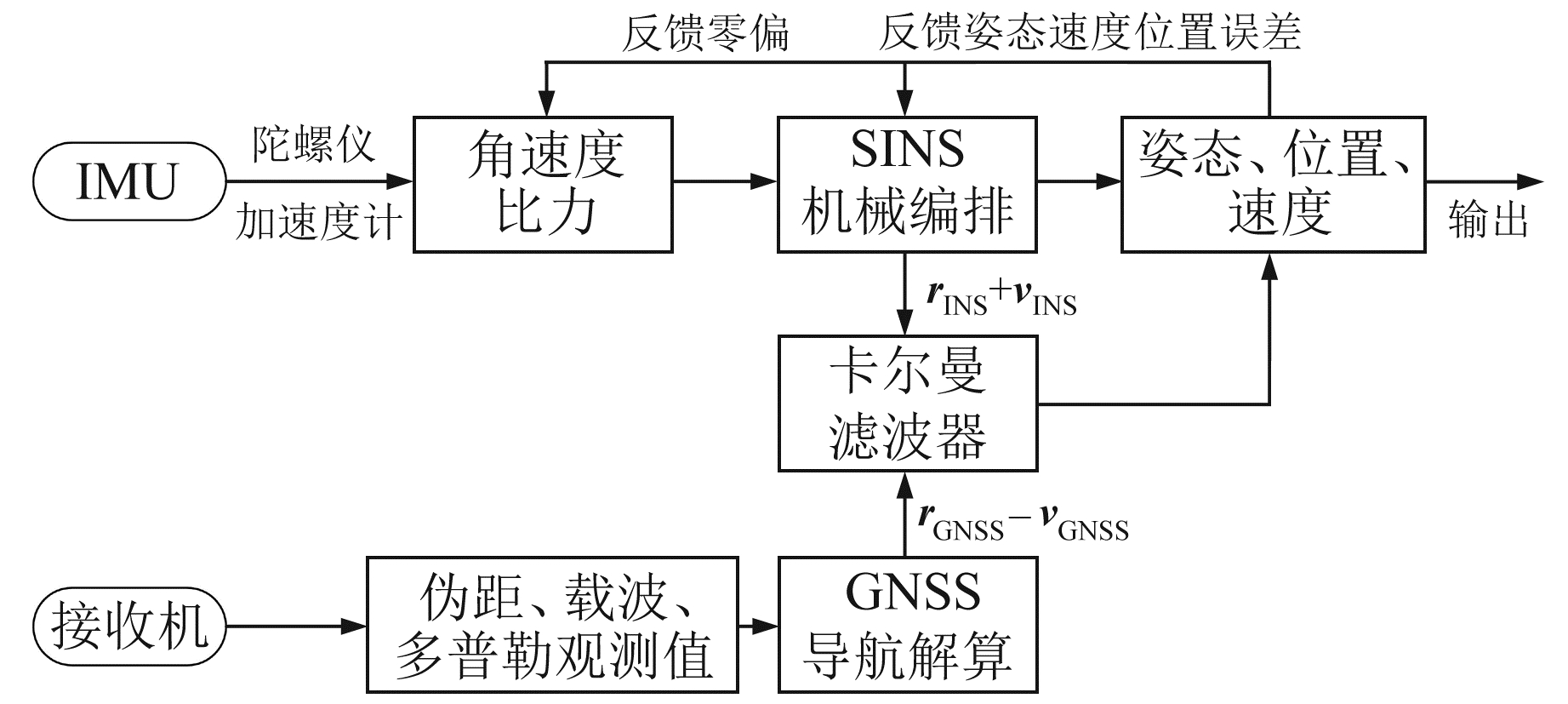图 1 松组合基本流程 Fig. 1 Basic flow chart of loosely coupled
1.2 自适应因子构造

 ${{\mathit{\boldsymbol{\tilde Z}}}_{k/k - 1}} = {\rm{ }}\mathit{\boldsymbol{Z}}{_k} - {\rm{ }}\mathit{\boldsymbol{H\hat X}}{}{_{k/k - 1}}$ (7)
 $\mathit{\boldsymbol{C}}{_k} = {\rm{ }}\mathit{\boldsymbol{H}}{_k}\mathit{\boldsymbol{P}}{_{k/k - 1}}\mathit{\boldsymbol{H}}_k^{\rm{T}} + {\rm{ }}\mathit{\boldsymbol{R}}{_k}$ (8)

 $\beta = {\rm{ }}\sqrt {\frac{{\mathit{\boldsymbol{Z}}_k^{\rm{T}}\mathit{\boldsymbol{Z}}{_k}}}{{{\rm{tr}}({\rm{ }}\mathit{\boldsymbol{C}}{_k})}}} {\rm{}}$ (9)

 $\alpha = \left\{ \begin{array}{l} {\rm{ }}1, \;\;\left| \beta \right| \le c\\ c/\left| \beta \right|, \;\;\left| \beta \right| > c \end{array} \right.$ (10)

 $\mathit{\boldsymbol{K}}{_k} = \left( {1/\alpha } \right){\rm{ }}\mathit{\boldsymbol{P}}{_{k/k - 1}}\mathit{\boldsymbol{H}}_k^{\rm{T}}{({\rm{ }}\mathit{\boldsymbol{H}}{_k}\mathit{\boldsymbol{P}}{_{k/k - 1}}\mathit{\boldsymbol{H}}_k^{\rm{T}} + {\rm{ }}\mathit{\boldsymbol{R}}{_k})^{ - 1}}$ (11)

2 数据采集图 2 车辆行驶轨迹 Fig. 2 Vehicle trajectory表 1 惯导仪器性能指标 Tab. 1 Performance index of inertial navigation instrument
3 算例分析 3.1 GNSS定位分析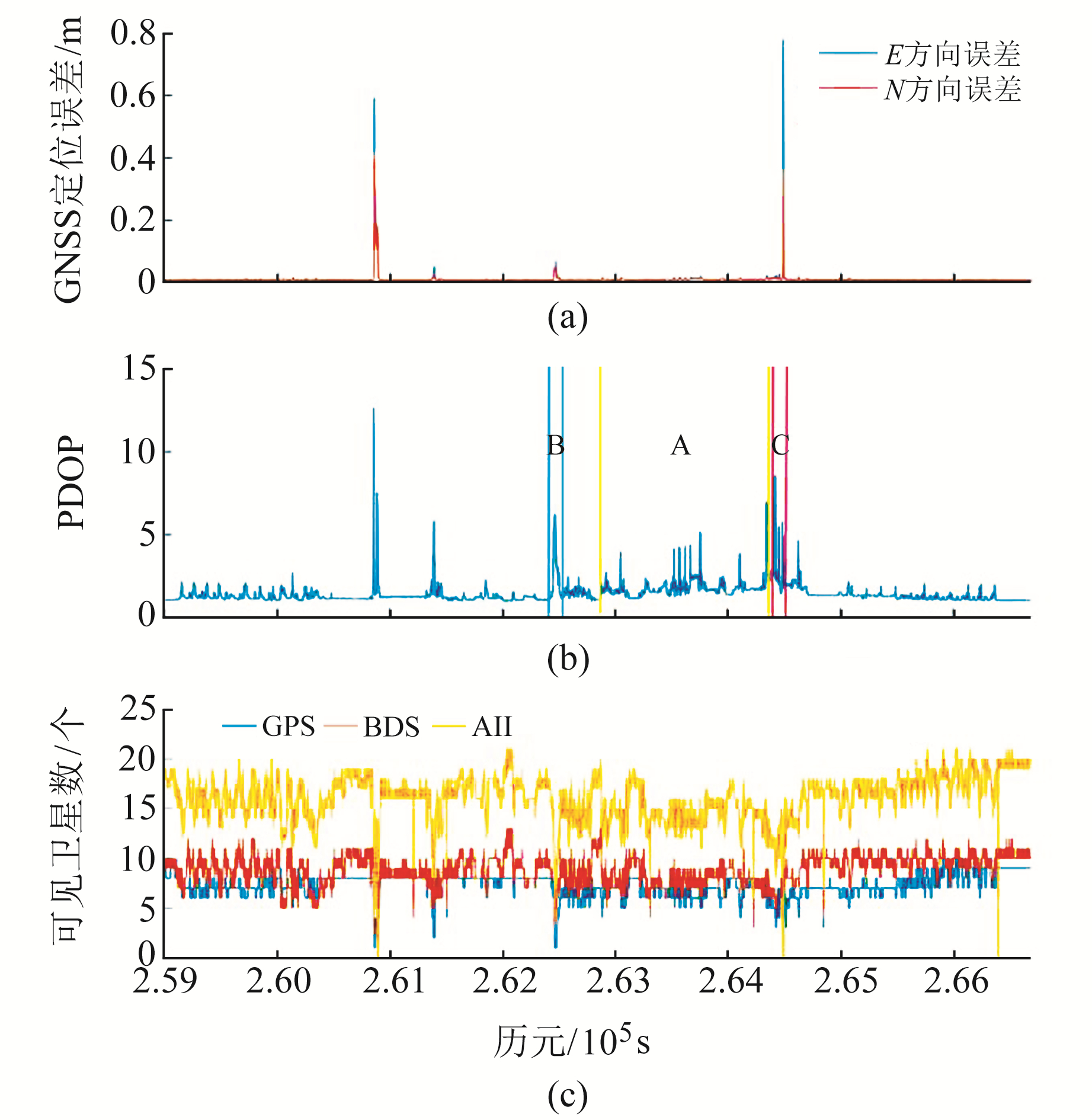图 3 GNSS定位误差、PDOP和可见卫星数 Fig. 3 GNSS positioning error, PDOP and number of visible satellites

3.2 组合导航算例 3.2.1 模拟卫星信号失锁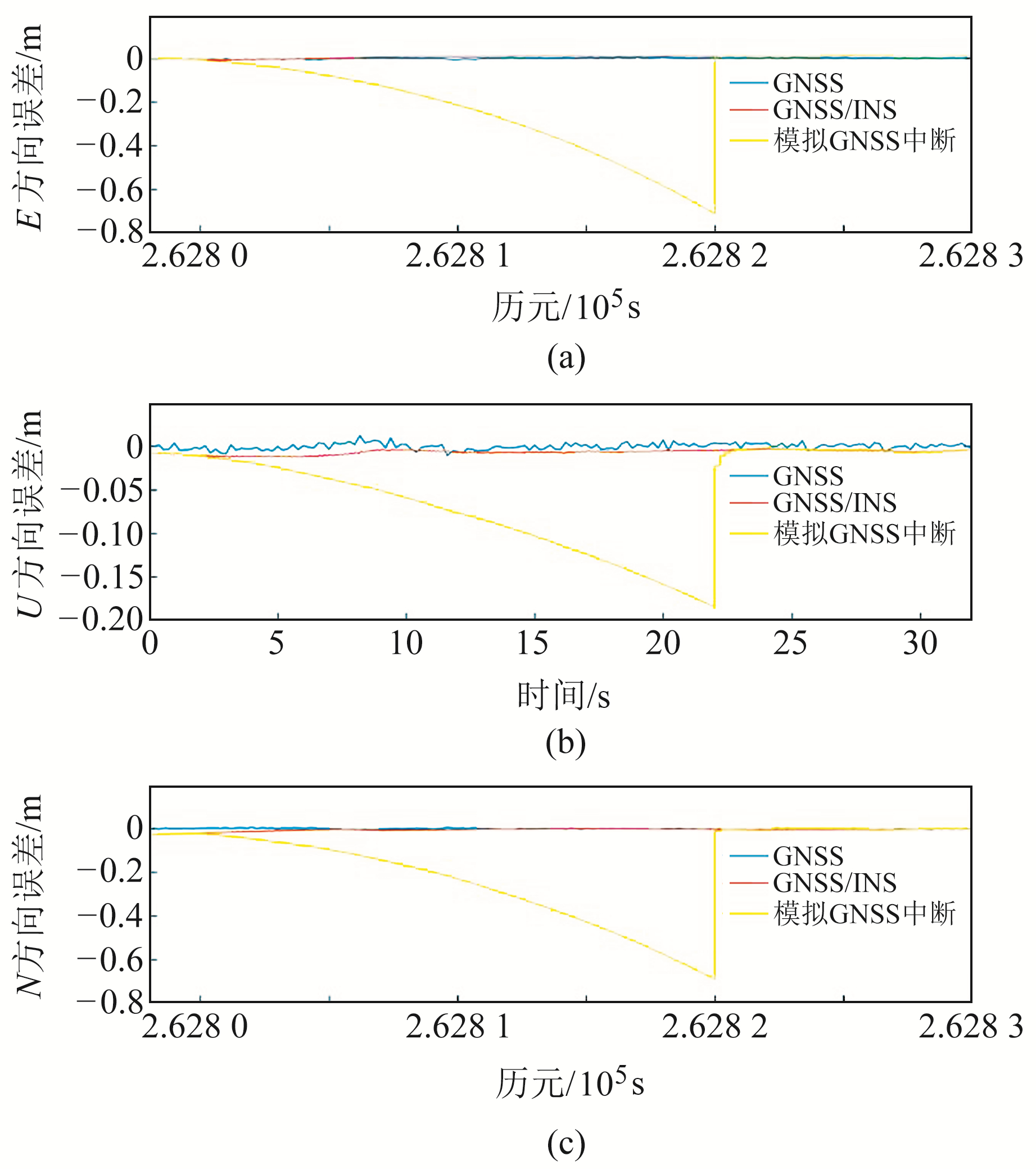图 4 模拟GNSS中断的组合导航 Fig. 4 Integrated navigation simulating GNSS interruption

3.2.2 卫星信号遮挡下的组合导航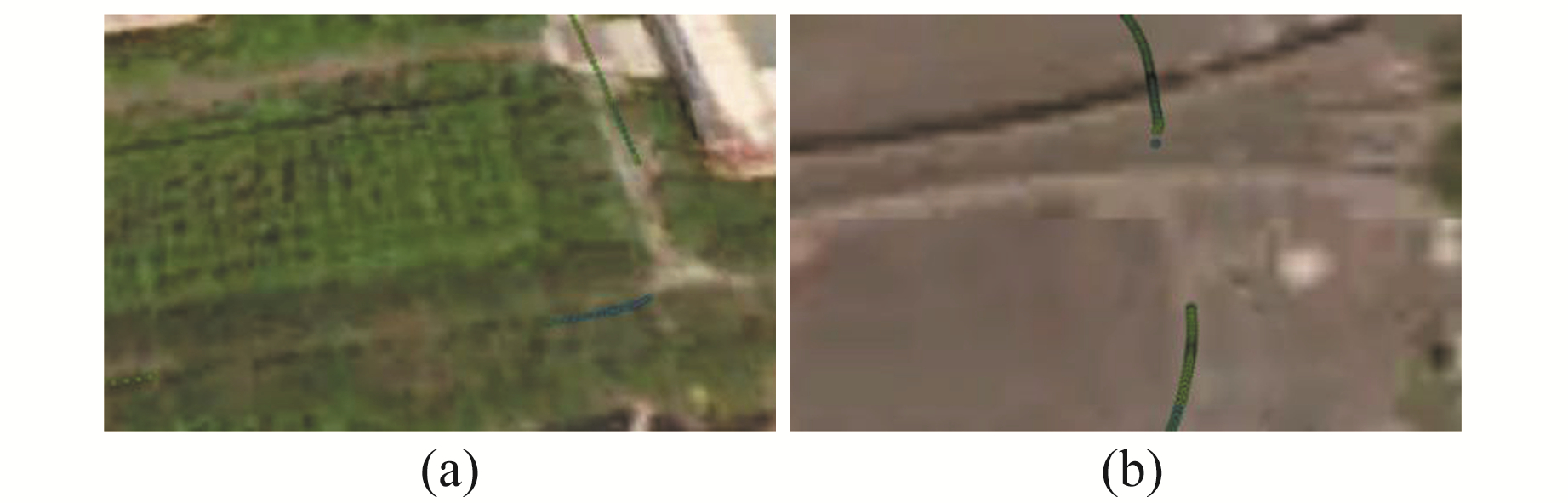图 5 GNSS定位结果 Fig. 5 GNSS positioning results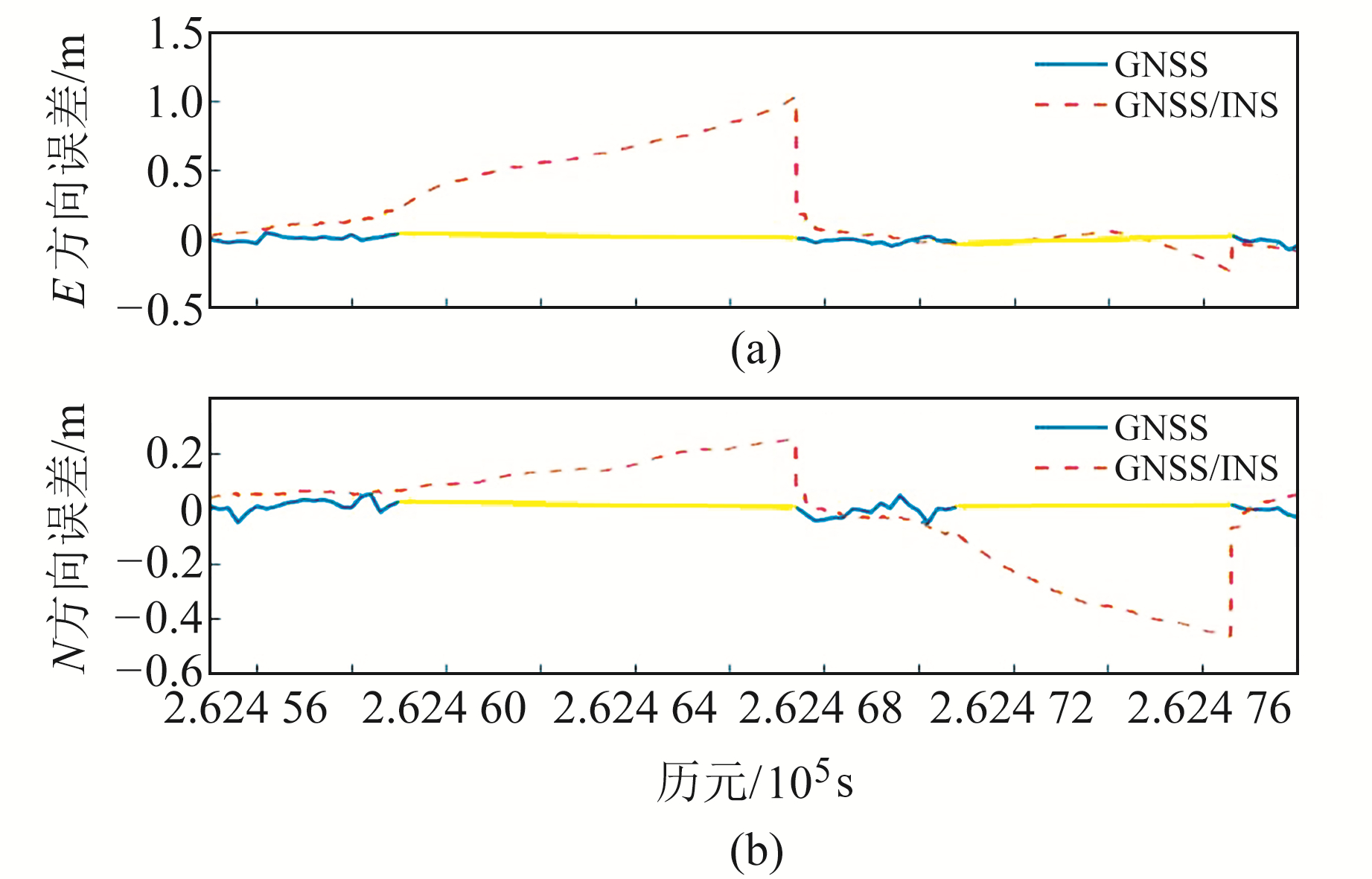图 6 树木密集区域GNSS导航与组合导航结果 Fig. 6 Results of GNSS navigation and integrated navigation in tree intensive area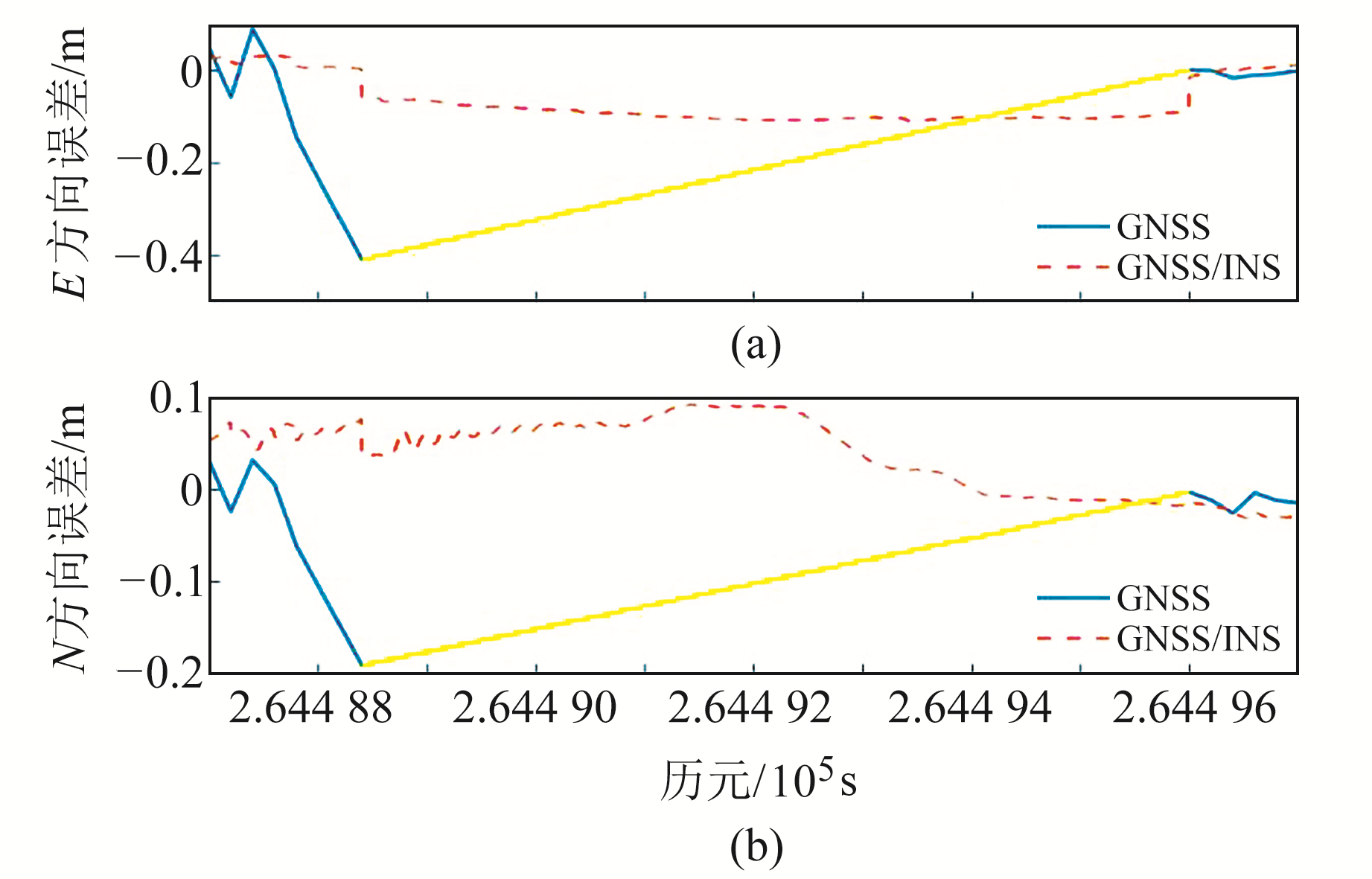图 7 高大门廊区域GNSS导航与组合导航结果 Fig. 7 Results of GNSS navigation and integrated navigation in high porch area

3.2.3 自适应卡尔曼滤波算法

A区域定位误差较小，但由于受到高楼、树木以及水面影响，其PDOP值仍然较大，部分时间段大于3，少数历元甚至大于4。由于接收机与其存在一定距离，卫星信号不至于被遮挡，但会导致较严重的多路径效应，使得信号受到一定影响。并且由于树木遮挡，使得只有高度角较高的卫星可见，卫星的空间几何构型和定位环境较差，导致定位误差波动较大图 8为该区域GNSS定位误差。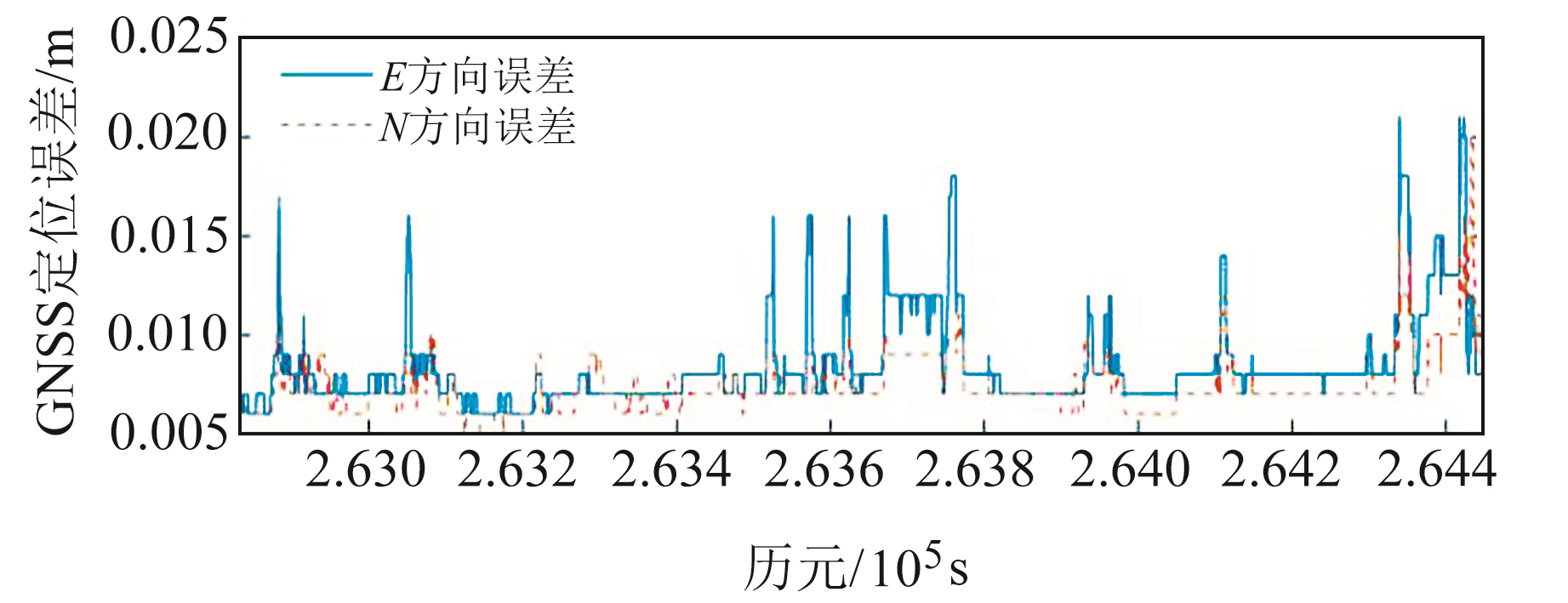图 8 A区域GNSS定位误差 Fig. 8 GNSS positioning errors in area A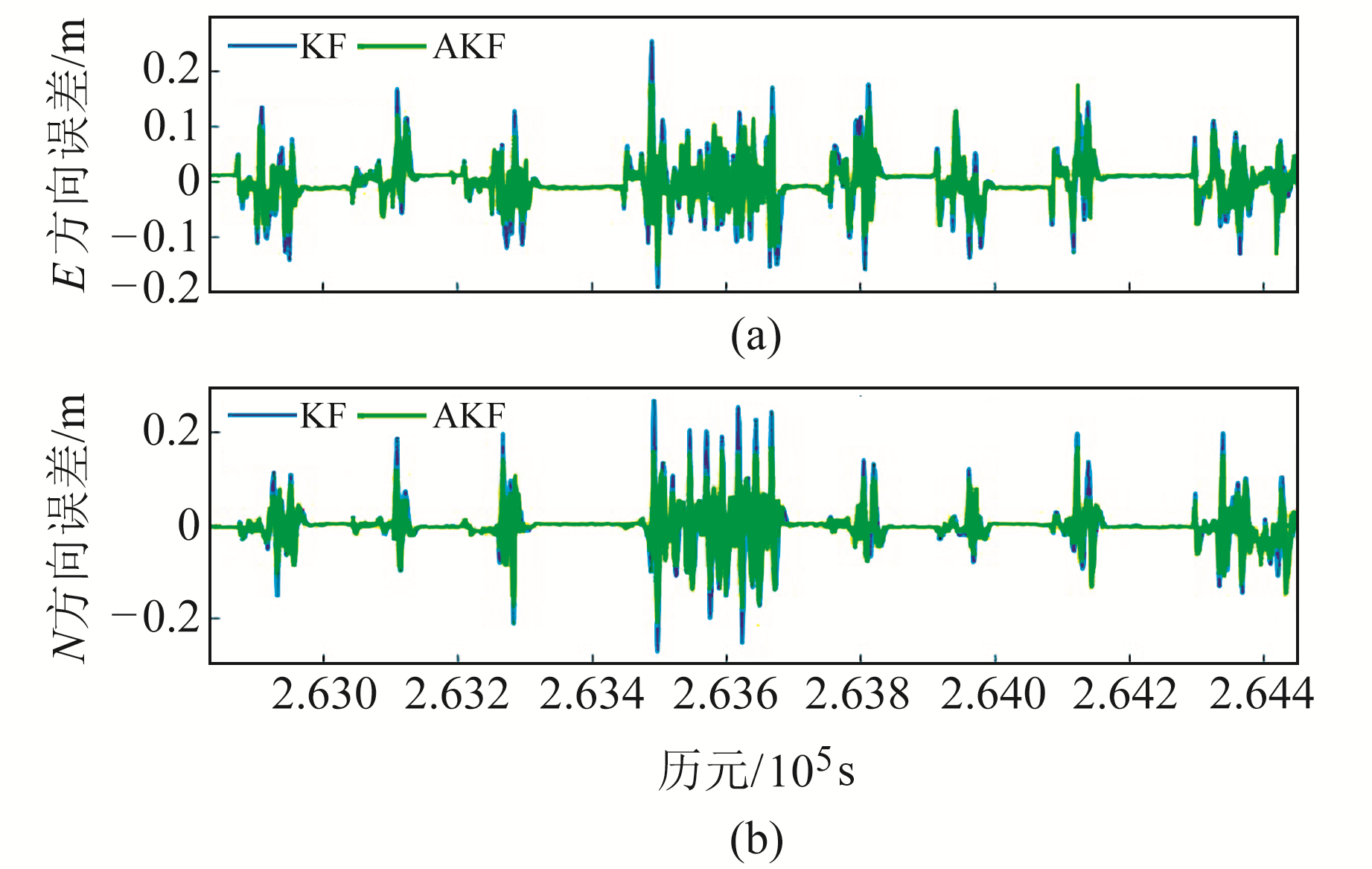图 9 KF、AKF位置误差 Fig. 9 KF, AKF position errors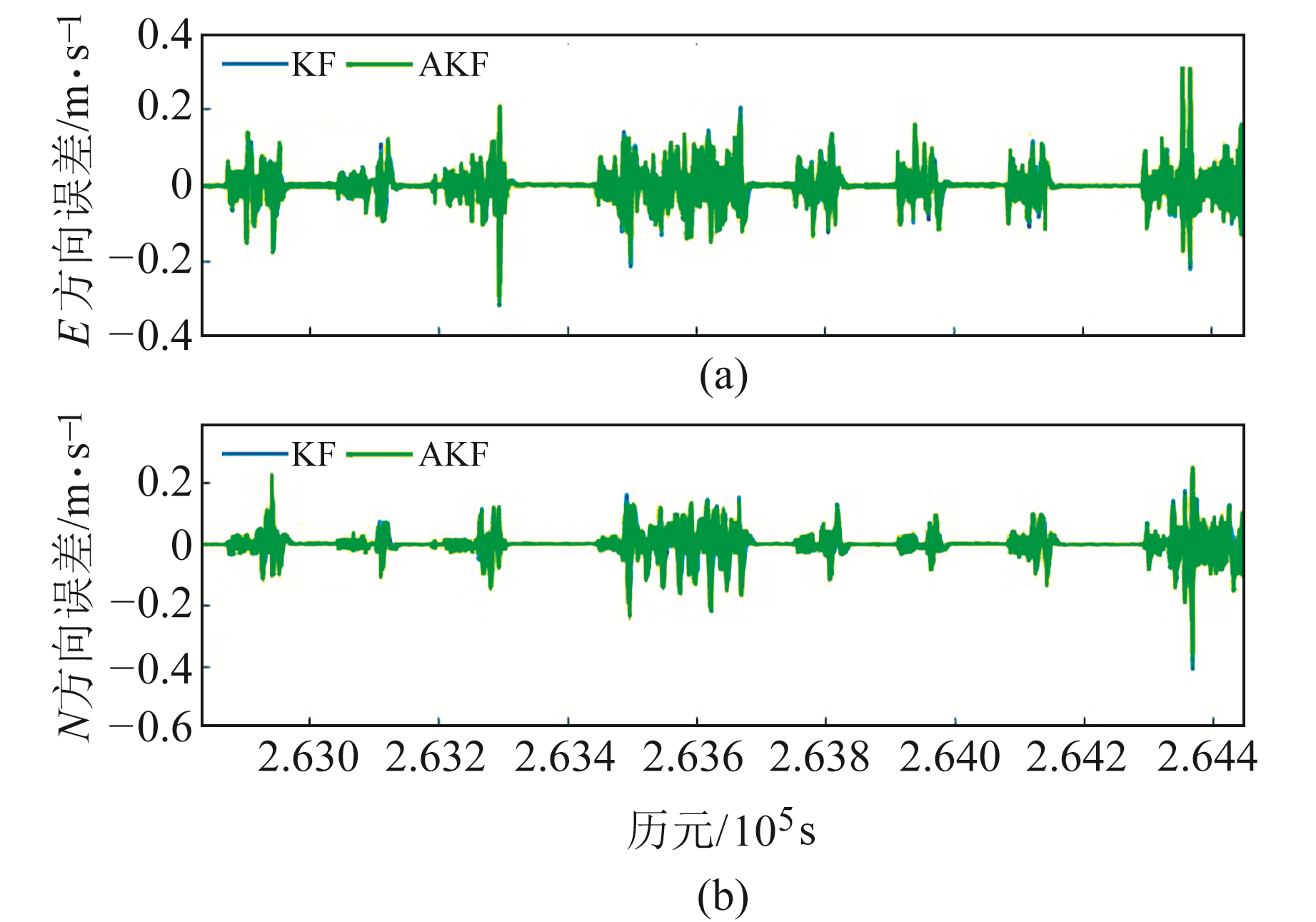图 10 KF、AKF速度误差 Fig. 10 KF, AKF velocity errors表 2 卡尔曼滤波算法位置结果对比 Tab. 2 Comparison of location results of Kalman filtering algorithm表 3 卡尔曼滤波算法速度结果对比 Tab. 3 Comparison of velocity results of Kalman filtering algorithm

4 结语

1) GNSS/INS松组合可在卫星信号失锁的短时间内提供较高精度的导航结果，组合导航系统方向累积误差的大小与卫星信号失锁时行驶方向的方位角有关，且车辆加减速时组合系统的可观测性得到提升。

2) 本文提出的自适应卡尔曼滤波算法可在卫星信号受多路径效应严重影响时显著提高GNSS/INS组合导航结果的稳定性和定位精度，相比于常规滤波算法精度可提高约30%。

  严恭敏. 捷联惯导算法及车载组合导航系统研究[D]. 西安: 西北工业大学, 2004 (Yan Gongmin. Research on SINS and Vehicle-Borne Integrated Navigation System[D]. Xi'an: Northwestern Polytechnical University, 2004) http://cdmd.cnki.com.cn/article/cdmd-10699-2004062822.htm (0)  秦永元, 张洪钺, 汪叔华. 卡尔曼滤波与组合导航原理[M]. 西安: 西北工业大学出版社, 2012 (Qin Yongyuan, Zhang Hongyue, Wang Shuhua. Kalman Filter and Integrated Navigation Principle[M]. Xi'an: Northwestern Polytechnical University Press, 2012) (0)  隋心, 李玉星, 沈佳琦, 等. 复杂环境下BDS/GPS紧组合高精度定位方法[J]. 导航定位学报, 2019, 7(1): 83-87 (Sui Xin, Li Yuxing, Shen Jiaqi, et al. High-Accurate Positioning Method of BDS/GPS Tight Combination under Complicated Environment[J]. Journal of Navigation and Positioning, 2019, 7(1): 83-87) (0)  姚卓, 章红平. 里程计辅助车载GNSS/INS组合导航性能分析[J]. 大地测量与地球动力学, 2018, 38(2): 206-210 (Yao Zhuo, Zhang Hongping. Performance Analysis on Vehicle GNSS/INS Integrated Navigation System Aided by Odometer[J]. Journal of Geodesy and Geodynamics, 2018, 38(2): 206-210) (0)  Chai D S, Chen G L, Wang S L, et al. Loosely Coupled GNSS/INS Integration Based on an Auto Regressive Model in a Data Gap Environment[J]. Acta Geodaetica et Geophysica, 2018, 53(4): 691-715 DOI:10.1007/s40328-018-0238-8 (0)  沈凯, 刘庭欣, 左思琪, 等. 复杂城市环境下GNSS/INS组合导航可观测度分析及鲁棒滤波方法[J]. 仪器仪表学报, 2020, 41(9): 252-261 (Shen Kai, Liu Tingxin, Zuo Siqi, et al. Observability Analysis and Robust Fusion Algorithms of GNSS/INS Integrated Navigation in Complex Urban Environment[J]. Chinese Journal of Scientific Instrument, 2020, 41(9): 252-261) (0)  邵梦杨, 郭磊, 王甫红. 城市典型环境下单频导航型GNSS接收机多路径误差特性分析[J]. 测绘通报, 2018(9): 1-7 (Shao Mengyang, Guo Lei, Wang Fuhong. Multipath Error Analysis of Single Frequency Navigation GNSS Receiver in Typical Urban Environment[J]. Bulletin of Surveying and Mapping, 2018(9): 1-7) (0)  Gao X L, Luo H Y, Ning B K, et al. RL-AKF: An Adaptive Kalman Filter Navigation Algorithm Based on Reinforcement Learning for Ground Vehicles[J]. Remote Sensing, 2020, 12(11): 1 704 DOI:10.3390/rs12111704 (0)  Ryan J G, Bevly D M. On the Observability of Loosely Coupled Global Positioning System/Inertial Navigation System Integrations with Five Degree of Freedom and Four Degree of Freedom Inertial Measurement Units[J]. Journal of Dynamic Systems, Measurement, and Control, 2014, 136(2): 021023 DOI:10.1115/1.4025985 (0)  杨元喜, 何海波, 徐天河. 论动态自适应滤波[J]. 测绘学报, 2001, 30(4): 293-298 (Yang Yuanxi, He Haibo, Xu Tianhe. Adaptive Robust Filtering for Kinematic GPS Positioning[J]. Acta Geodaetica et Cartographic Sinica, 2001, 30(4): 293-298) (0)  徐天河, 杨元喜. 改进的Sage自适应滤波方法[J]. 测绘科学, 2000, 25(3): 22-24 (Xu Tianhe, Yang Yuanxi. The Improved Method of Sage Adaptive Filtering[J]. Developments in Surveying and Mapping, 2000, 25(3): 22-24) (0)  柴大帅. 多星座GNSS/INS组合导航理论与方法研究[D]. 徐州: 中国矿业大学, 2020 (Chai Dashuai. Study on the Theory and Method in Integrated Navigation of Multi-Constellation GNSS/INS[D]. Xuzhou: China University of Mining and Technology, 2020) http://cdmd.cnki.com.cn/Article/CDMD-10290-1020962693.htm (0)
Research on GNSS/INS Integrated Navigation Algorithm in Complex Urban Environments
WANG Fu1HAN Baomin1HU Liangliang2     MENG Hao1     GUO Zhenhua3
1. School of Civil and Architectural Engineering, Shandong University of Technology, 266 West-Xincun Road, Zibo 255000, China;
2. College of Geodesy and Geomatics, Shandong University of Science and Technology, 579 Qianwangang Road, Qingdao 266590, China;
3. Shandong Gold Mining (Linglong) Co Ltd, 999 Huangshui Road, Zhaoyuan 265400, China
Abstract: Aiming at the problem of susceptibility to satellite signals in GNSS vehicle navigation in urban environments, we use the GNSS/INS combined algorithm to improve positioning performance of urban vehicles in complex environments. Based on actual measurement GNSS data from the urban environment, we evaluate and analyze the positioning results, and use the conventional Kalman filtering algorithm of the GNSS/INS combination to realize the navigation of the satellite lock-out area. At the same time, we propose an adaptive Kalman filtering algorithm based on innovation, which can effectively enhance the navigation and positioning capabilities of vehicles in areas with fewer satellites and severe signal interference. This method uses the relationship between measurement and prediction to construct an adaptive factor to improve positioning accuracy. The results show that the conventional Kalman filter can guarantee sub-meter navigation accuracy when the satellite signal is out of lock in 20 s. For satellite signals with severely interference, the positioning accuracy of the adaptive Kalman filtering algorithm is increased by 30% when compared with the conventional Kalman filter. The adaptive Kalman filtering algorithm can meet the needs of high-precision and high-reliability vehicle navigation and positioning services in the complex urban environment.
Key words: urban environment; Kalman filter; GNSS/INS; loosely coupled